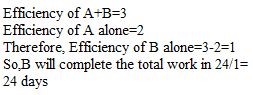# PROBLEMS ON TIME AND WORK-LERAN SERIES

Hi Bankersdaily Aspirants,
Learning is a step by step process and you should gradually progress in that to ace the process. As you all know we have started a new Learn Series to help with your preparations for various exams like IBPS PO , IBPS RRB Scale I officer , IBPS RRB Office Assistant , IBPS Clerk , IBPS SO , SBI PO , SBI Clerk , SBI SO and many other exams in this type. Nevertheless this series will also contribute you all the tips and tricks to every miscellaneous sections and also all the important topics which are asked in the exams. If you haven’t looked at the Learn series , Please Click the below link to learn the new concepts.

Also we are coming daily with different topics and if you haven’t checked our latest article on the Problems based on Profit and Loss Part 1,Please Click the link below

### INTRODUCTION TO TIME AND WORK:

There will be 1 or 2 Questions from this topic in all IBPS Exams.This topic is quite easy,Since Time and Work can be solved in Partial Method in our article we use LCM method to solve the problem in order to reduce the time consumption.There are 8 Basic types in Time and Work.Let us discuss each type with an example.

Before getting into the types let us see 3 important terms associated with Time and Work,

1st Term:Work = The total work which has to be completed

Example:It is not just the work,it may be eating ice creams,completing assignment it may be any work.

If Total Work is not given question then Total Work is LCM of Given numbers

2nd Term:Time=The time taken to complete the work it may be in Hours,Minute, Days,Months and Years

3rd Term Efficiency=Total Work/Time

Efficiency is nothing but  Per day Capacity of the individual

Let us look each type with example:

## #.1.TYPE 1:### PROBLEM BASED ON FINDING TOGETHER WORK:

In this type,we will be given the individual Capacity and we are asked to find the total working capacity.

1)A can complete the 15 days while B can do the same work in 30 days.Find in how many days they will take to complete the work if they work together?

Explanation

Here Total Work=LCM ( 15,30)=30

A’s Efficiency=Total Work /Time Taken = 30/15=2

So A can complete 2 task/day

B’s Efficiency=Total Work/Time Taken = 30/30=1

So B  can Complete 1 task/day

When A and B combines together they can complete 3 task per day (2 + 1)

Then Time Taken=Total work/Efficiency

= 30/3

Time Taken to Complete the task when both A & B work together=10 Days

## #. 2.TYPE 2:### PROBLEMS BASED ON FINDING INDIVIDUAL WORK:

In this type we will be given together working capacity and one of their individual working capacity.

1)A works alone and complete the work in 12 days and when A and B work together they complete the work in 8 days then find in how many days B alone completes the work?

Explanation

Given that,

A+B completes the work in 8 days

A  Alone Completes the work in 12 days

As I already said Total Work is LCM of given numbers

Therefore Total Work =LCM(8,12)=24

Efficiency of A and B when they work together=24/8=3

Efficiency of A (when Works alone)=24/12=2

It is Clear that B’s Efficiency=1## #.3.TYPE 3:

### PROBLEMS BASED ON PARTIAL WORK:

This is again sub divided into 2 types:

1)Somebody will leave the group

2)Somebody will join the group

1)Somebody leave the group:In this type,initially the people works together and after some day one of the them will leave the group.

1)A can Complete the total work in 25 days and B can Complete the total work in 15 days,Initially they both work together and After 5 days A Left,Find in how many days B alone take to complete  the remaining work?

Explanation

Total Work=LCM (25,15)=75

When A & B work together:

Their together Efficiency=5+3 = 8 task/day

Therefore for 5 days they will complete 8*5=40 task

Now,B only left,B can Complete 5 task/day so Remaining 35 task is completed by B in 7 days

2)Someone Joins the group:1)A can complete the work in 4 days and B can complete the work in 5 days.With the help of C,when A,B and C works together they can complete the work in 2 days.Find in how many days C alone Complete the work?

Explanation

Total Work=LCM (4,5)=20

Efficiency of A=20/4=5 work/day

Efficiency of B=20/5=4 work/day

Given that,When A,B and C work together they complete the task in 2 days

We know that A+B can Complete the 9 task/day therefore Remaining 1 task is done by C

So,C complete’s the total work in 20/1=20 days